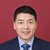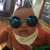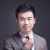Go语言的设计者计划设计一门简单、高效、安全和 并发的语言。这门语言简单到甚至不需要有一个符号表来进行词法分析。它可以快速地编译；整个工程的编译时间在秒以下的情况是常事。它具备垃圾回收功能，因 此从内存的角度是安全的。它进行静态类型检查，并且不允许强制类型转换，因而对于类型而言是安全的。同时语言还内建了强大的并发实现机制。

## 阅读Go

Go的语法传承了与C一样的风格。程序由函数组成，而函数体是一系列的语句序列。一段代码块用花括号括起来。这门语言保留有限的关键字。表达式使用 同样的中缀运算符。语法上并 无 太多出奇之处。

Go语言的作者在设计这一语言时坚持一个单一的指导原则：简单明了至上。一些新的语法构件提供了简明地表达一些约定俗成的概 念的方式，相较之下用C表达显得冗长。而其他方面则是针对几十年的使用所呈现出来的一些不合理的语言选择作出了改进。

### 变量声明

`var sum int // 简单声明var total int = 42 // 声明并初始化`

`int* a, b;`

`var a, b *int`

`var label = "name"`

`name := "Samuel"`

### 条件语句

Go语言当中的条件句与C当中所熟知的if-else构造一样，但条件不需要被打包在括号内。这样可以减少阅读代码时的视觉上的混乱。

`result := someFunc();if result > 0 {	/* Do something */} else {	/* Handle error */}`

`if result := someFunc(); result > 0 { 	/* Do something */} else {	/* Handle error */}`

### 分支语句

`int result;switch (byte) { case 'a': case 'b':   {     result = 1     break   } default:   result = 0}`

`var result intswitch byte {case 'a', 'b':  result = 1default:  result = 0}`

`int result = calculate();if (result < 0) {  /* negative */} else if (result > 0) {  /* positive */} else {  /* zero */}`

`switch result := calculate(); true {case result < 0:  /* negative */case result > 0:  /* positive */default:  /* zero */}`

`switch result := calculate(); {case result < 0:  /* negative */case result > 0:  /* positive */default:  /* zero */}`

### 循环

Go只有一个关键字用于引入循环。但它提供了除do-while外C语言当中所有可用的循环方式。

#### 条件

`for a > b { /* ... */ }`

#### 初始，条件和步进

`for i := 0; i < 10; i++ { /* ... */ }`

#### 范围

range语句右边的表达式必须是array，slice，string或者map， 或是指向array的指针，也可以是channel。

`for i := range "hello" { /* ... */ }`

#### 无限循环

`for { /* ever */ }`

### 函数

`int add(int a, b) { return a + b }`

`func add(a, b int) int { return a + b }`

#### 多返回值

`func divide(a, b int) (int, int) {  quotient := a / b  remainder := a % b  return quotient, remainder}`

`func divide(a, b int) (quotient, remainder int) {  quotient = a / b  remainder = a % b  return}`

`if result, ok := moreMagic(); ok {  /* Do something with result */}`

#### 匿名函数

`func makeAdder(x int) (func(int) int) {  return func(y int) int { return x + y }}func main() {  add5 := makeAdder(5)  add36 := makeAdder(36)  fmt.Println("The answer:", add5(add36(1))) //=> The answer: 42}`

### 基本类型

#### 数组和切片

Go语言当中的数组不是像C语言那样动态的。它们的大小是类型的一部分，在编译时就决定了。数组的索引还是使用的熟悉的C语法(如 a[i])，并且与C一样，索引是由0开始的。编译器提供了内建的功能在编译时求得一个数组的长度 (如 len(a))。如果试图超过数组界限写入，会产生一个运行时错误。

Go还提供了切片（slices），作为数组的变形。一个切片(slice)表示一个数组内的连续分段，支持程序员指定底层存储的明确部分。构建一 个切片 的语法与访问一个数组元素类似：

`/* Construct a slice on ary that starts at s and is len elements long */s1 := ary[s:len]/* Omit the length to create a slice to the end of ary */s2 := ary[s:]/* Slices behave just like arrays */s == ary[s] //=> true// Changing the value in a slice changes it in the arrayary[s] = 1s = 42ary[s] == 42 //=> true`

`/* Move the start of the slice forward by one, but do not move the end */s2 = s2[1:]/* Slices can only move forward */s2 = s2[-1:] // this is a compile error`

`a := [...]int{1,2,3,4,5} // The ... means "whatever length the initializer has"len(a) //=> 5/* Slice from the middle */s := a[2:4] //=> [3 4]len(s), cap(s) //=> 2, 3/* Grow the slice */s = s[0:3] //=> [3 4 5]len(s), cap(s) //=> 3, 3/* Cannot grow it past its capacity */s = s[0:4] // this is a compile error`

`/* literal */s1 := []int{1,2,3,4,5}/* empty (all zero values) */s2 := make([]int, 10) // cap(s2) == len(s2) == 10`

#### Map类型

`m := make(map[string] int) // A mapping of strings to ints/* Store some values */m["foo"] = 42m["bar"] = 30/* Read, and exit program with a runtime error if key is not present. */x := m["foo"]/* Read, with comma-ok check; ok will be false if key was not present. */x, ok := m["bar"]/* Check for presence of key, _ means "I don't care about this value." */_, ok := m["baz"] // ok == false/* Assign zero as a valid value */m["foo"] = 0;_, ok := m["foo"] // ok == true/* Delete a key */m["bar"] = 0, false_, ok := m["bar"] // ok == false`

## 面向对象

Go语言支持类似于C语言中使用的面向对象风格。数据被组织成structs，然后定义操作这些structs的函数。类似于Python，Go语 言提供 了定义函数并调用它们的方式，因此语法并不会笨拙。

### Struct类型

`type Point struct {  x, y float64}`

`var p *Point = new(Point)p.x = 3p.y = 4`

`var p1 Point = Point{3,4}  // Valuevar p2 *Point = &Point{3,4} // Pointer`

### 方法

`func (self Point) Length() float {  return math.Sqrt(self.x*self.x + self.y*self.y);}`

`p := Point{3,4}d := p.Length() //=> 5`

`/* Note the receiver is *Point */func (self *Point) Scale(factor float64) {  self.x = self.x * factor  self.y = self.y * factor}`

`p.Scale(2);d = p.Length() //=> 10`

### 接口

`type Writer interface {  Write(p []byte) (n int, err os.Error)}`

Go当中接口的运作方式支持开发者在编写程序的时候发现程序的类型。如果几个对象间存在公共行为，而开发者想要抽象这种行为，那么它就可以创建一个 接口并使用它。

`// Somewhere in some code:type Widget struct {}func (Widget) Frob() { /* do something */ }// Somewhere else in the code:type Sprocket struct {}func (Sprocket) Frob() { /* do something else */ }/* New code, and we want to take both Widgets and Sprockets and Frob them */type Frobber interface {  Frob()}func frobtastic(f Frobber) { f.Frob() }`

`interface {}`

### 继承

Go语言不支持继承，至少与大多数语言的继承不一样。并不存在类型的层次结构。相较于继承，Go鼓励使用组合和委派，并为此提供了相应的语法甜点使 其更容易接受。

`type Engine interface {  Start()  Stop()}type Car struct {  Engine}`

`func GoToWorkIn(c Car) {  /* get in car */  c.Start();  /* drive to work */  c.Stop();  /* get out of car */}`

`type Base struct {}func (Base) Magic() { fmt.Print("base magic") }func (self Base) MoreMagic() {   self.Magic()  self.Magic()}type Foo struct {  Base}func (Foo) Magic() { fmt.Print("foo magic") }`

`f := new(Foo)f.Magic() //=> foo magicf.MoreMagic() //=> base magic base magic`

## 并发

Go的作者选择了消息传递模型来作为推荐的并发编程方法。该语言同样支持共享内存，然后作者自有道理：

### Go例程

Goroutine是轻量级的并行程序执行路径，与线程，coroutine或者进程类似。然而，它们彼此相当不同，因此Go作者决定给它一个新的 名字并 放弃其它术语可能隐含的意义。

`go DoThis() // but do not wait for it to complete`

`go func() {  for { /* do something forever */ }}() // Note that the function must be invoked`

### 通道类型

`/* Creating a channel uses make(), not new - it was also used for map creation */ch := make(chan int)/* Sending a value blocks until the value is read */ch <- 4/* Reading a value blocks until a value is available */i := <-ch`

`ch := make(chan int)go func() {  result := 0  for i := 0; i < 100000000; i++ {    result = result + i  }  ch <- result}()/* Do something for a while */sum := <-ch // This will block if the calculation is not done yetfmt.Println("The sum is:", sum)`

channel的阻塞行为并非永远是最佳的。该语言提供了两种对其进行定制的方式：

1. 程序员可以指定缓冲大小——想缓冲的channel发送消息不会阻塞，除非缓冲已满，同样从缓冲的channel读取也不会阻塞，除非缓 冲是空的。
2. 该语言同时还提供了不会被阻塞的发送和接收的能力，而操作成功是仍然要报告。
`/* Create a channel with buffer size 5 */ch := make(chan int, 5)/* Send without blocking, ok will be true if value was buffered */ok := ch <- 42/* Read without blocking, ok will be true if a value was read */val, ok := <-ch`

## 包

Go提供了一种简单的机制来组织代码：包。每个文件开头都会声明它属于哪一个包，每个文件也可以引入它所用到的包。任何首字母大写的名字是由包导出 的，并可以被其它的包所使用。

`package geometryimport "math"/* Point is capitalized, so it is visible outside the package. */type Point struct {  /* the fields are not capitalized, so they are not visible     outside of the package */  x, y float64 }/* These functions are visible outside of the package */func (self Point) Length() float64 {  /* This uses a function in the math package */  return math.Sqrt(self.x*self.x + self.y*self.y)}func (self *Point) Scale(factor float64) {  self.setX(self.x * factor)  self.setY(self.y * factor)}/* These functions are not visible outside of the package, but can be   used inside the package */func (self *Point) setX(x float64) { self.x = x }func (self *Point) setY(y float64) { self.y = y }`

## 缺失

Go语言的作者试图将代码的清晰明确作为设计该语言作出所有决定的指导思想。第二个目标是生产一个编译速度很快的语言。有了这两个标准作为方向，来 自其它语言的许多特性就不那么适合了。许多程序员会发现他们最爱的语言特性在Go当中不存在，确实，有很多人也许会觉得Go语言由于缺乏其它语言所共有的 一些特性，还不太可用。

1#### 引用来自“红猎人”的帖子

加点注解就更好了

00#### 引用来自“红猎人”的帖子

加点注解就更好了

00Go关注的领域是什么？云风对这个Go赞赏有加，哎，有时间去看看